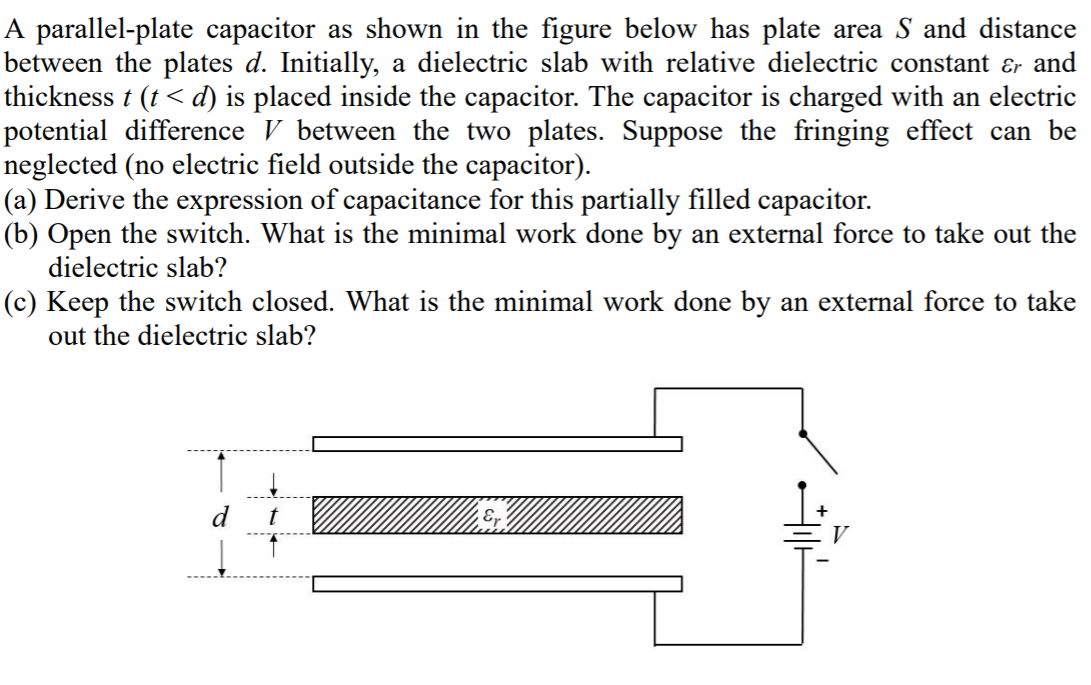Home / Answered Questions / Other / a-parallel-plate-capacitor-as-shown-in-the-figure-below-has-plate-area-s-and-distance-between-the-pl-aw176

# (Solved): A Parallel-plate Capacitor As Shown In The Figure Below Has Plate Area S And Distance Between The Pl...A parallel-plate capacitor as shown in the figure below has plate area S and distance between the plates d. Initially, a dielectric slab with relative dielectric constant er and thickness t (t<d) is placed inside the capacitor. The capacitor is charged with an electric potential difference V between the two plates. Suppose the fringing effect can be neglected (no electric field outside the capacitor). (a) Derive the expression of capacitance for this partially filled capacitor. (b) Open the switch. What is the minimal work done by an external force to take out the dielectric slab? (c) Keep the switch closed. What is the minimal work done by an external force to take out the dielectric slab?

We have an Answer from Expert